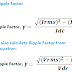The phenomenon of Ripple or Ripple Factor in electronics as well as electrical is a very important topic. So, you must have knowledge about that. There are some important terms also you should know such as Ripple Voltage, Ripple Current, Ripple Component, etc. Actually, the Ripple is coming under discussion when we converting the Alternating Current(AC) into Direct Current(DC) using any type of rectifier circuit. There is no rectifier circuit that can give pure DC output without any ripple.
So, our main discussion topic is how ripple comes and how to eliminate or reduce ripple.

## What is Ripple Factor?

The unwanted AC components present in the DC supply come from the rectifier output is called Ripple.

The rectifier is an electrical or electronic circuit that converts the alternating current into the direct current. A rectifier circuit cannot provide pure DC supply, some unwanted AC components also are there. During the conversion of AC to DC, Ripples produces and it comes in the rectifier output with the DC supply.

Generally, the production of ripple is dependent upon different factors such as the characteristics of rectifier components such as Diode, SCR. The nature of the input alternating supply also impacts the production of ripple.

To calculate or know the amount of produced ripple mathematically, the term 'Ripple Factor' is used. So, if we see the definition of Ripple factor is, the ratio of RMS value of input AC component of the rectifier to DC component from the output of the rectifier is called Ripple Factor. Or, in simple words, the ratio of RMS AC component to RMS DC component is called Ripple Factor.

## Significance of Ripple Factor and Problems

Ripple Factor indicates the efficiency of a rectifier circuit. If the rectifier circuit generates more ripples then the efficiency will be low, so less ripple generation means high efficiency. Ripple factor also indicates the nature of output DC supply. By, calculating the ripple factor, we can use the appropriate value of the filter component to filter the unfiltered DC that comes from the rectifier circuit.

The bad effects of Ripple factor are,

1. It causes noise and distortion in DC circuits.

2. It causes unwanted power losses in electrical circuits.

3. It causes power surges, overheating in circuits.

4. It can affect the operation of load connected to it. Even it may damage a very sensitive load.

5. The unfiltered output from a rectifier that have ripples may provide the lower voltage than the output that is filtered or have no ripple.

## Ripple Factor Formula, Equation, and Calculation

Ripple Factor can be calculated from the RMS Value and average values of the Rectifier input and output components. Before going to know the formula of ripple factor you should know these,

Irms = Alternating component in the output load current
Vrms = Alternating component in the output DC voltage
Idc = the value of pure DC current
Vdc = the value of pure DC voltage

Now, let's see the formula.

## Ripple Factor of Half Wave Rectifier

The Half Wave Rectifier is made up using a single PN Junction Diode. It only allows either positive half cycles or negative half cycles. The main disadvantage of the half-wave rectifier is that the output DC voltage got lower half than the input AC voltage. This happens due to the allowance of only one half cycle.

So, from the above equation, you can see, the value of the ripple factor of half wave rectifier is 1.21

## Ripple Factor of Full Wave Rectifier

The full wave rectifier circuit is made up using two PN Junction Diodes and a center-tapped transformer. The full wave rectifier circuit is designed in such a way that, it allows the positive half cycle and also negative half cycles by converting into the positive half cycle. The main advantage of the full wave rectifier circuit is, here the output voltage is not reduced like a half wave rectifier.

So, from the above equation, you can see, the value of the ripple factor of full wave rectifier is 0.48

## Ripple Factor of Bridge Rectifier

Bridge Rectifier Circuit is made up using four PN Junction Diodes. It also converts both positive and negative half-cycles like a center-tapped full wave rectifier circuit. The main advantage of the bridge rectifier circuit is, it does not require any center-tapped transformer. The efficiency of the bridge rectifier circuit is also very high over other rectifier circuits.

From the above equation, you can see the value of the ripple factor of bridge rectifier is 0.48 which is the same as the full wave rectifier.

## Reduction or Elimination of Ripple Factor

The ripple factor can be reduced or eliminated by connecting an electronic filter circuit to the output of the rectifier circuit. This filter circuit is made up using a Capacitor or Inductor. Generally, capacitors are mostly used to reduce the ripple. The capacitor is connected in parallel to the circuit. The main advantage of using a capacitor is, it does not make the voltage drop. On the other hand, if we use inductor, it causes a voltage drop and lowers the output voltage. An inductor filter blocks the ripples whereas an capacitor filter bypass the ripples to the ground.

What is Ripple Factor of Rectifier? Formula ExplainedReviewed by Author on July 14, 2021 Rating: 5